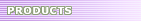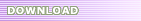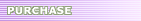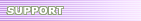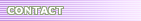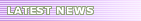# Java Code Conventions - Programming Practices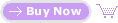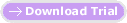You can read and download this coding style guide, coding standard, code convention, code guideline, manual or reference from here for free at your own risk. All trademarks, registered trademarks, product names and company names or logos mentioned herein are the property of their respective owners.

## 10 - Programming Practices

Don't make any instance or class variable public without good reason. Often, instance variables don't need to be explicitly set or gotten-often that happens as a side effect of method calls.

One example of appropriate public instance variables is the case where the class is essentially a data structure, with no behavior. In other words, if you would have used a `struct` instead of a class (if Java supported `struct)`, then it's appropriate to make the class's instance variables public.

### 10.2 Referring to Class Variables and Methods

Avoid using an object to access a class (static) variable or method. Use a class name instead. For example:

```classMethod();             //OK
AClass.classMethod();      //OK
anObject.classMethod();    //AVOID!
```

### 10.3 Constants

Numerical constants (literals) should not be coded directly, except for -1, 0, and 1, which can appear in a `for` loop as counter values.

### 10.4 Variable Assignments

Avoid assigning several variables to the same value in a single statement. It is hard to read. Example:

```fooBar.fChar = barFoo.lchar = 'c'; // AVOID!
```

Do not use the assignment operator in a place where it can be easily confused with the equality operator. Example:

```if (c++ = d++) {        // AVOID! (Java disallows)
...
}
```

should be written as

```if ((c++ = d++) != 0) {
...
}
```

Do not use embedded assignments in an attempt to improve run-time performance. This is the job of the compiler. Example:

```d = (a = b + c) + r;        // AVOID!
```

should be written as

```a = b + c;
d = a + r;
```

### 10.5 Miscellaneous Practices

#### 10.5.1 Parentheses

It is generally a good idea to use parentheses liberally in expressions involving mixed operators to avoid operator precedence problems. Even if the operator precedence seems clear to you, it might not be to others-you shouldn't assume that other programmers know precedence as well as you do.

```if (a == b && c == d)     // AVOID!
if ((a == b) && (c == d)) // RIGHT
```

#### 10.5.2 Returning Values

Try to make the structure of your program match the intent. Example:

```if (booleanExpression) {
return true;
} else {
return false;
}
```

```return booleanExpression;
```

Similarly,

```if (condition) {
return x;
}
return y;
```

should be written as

```return (condition ? x : y);
```

#### 10.5.3 Expressions before '?' in the Conditional Operator

If an expression containing a binary operator appears before the `?` in the ternary `?: `operator, it should be parenthesized. Example:

```(x >= 0) ? x : -x;
```

Use `XXX` in a comment to flag something that is bogus but works. Use `FIXME` to flag something that is bogus and broken.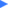Formatting Java source code by hand?  Try SourceFormatX Java Code Formatter to format your Java code!